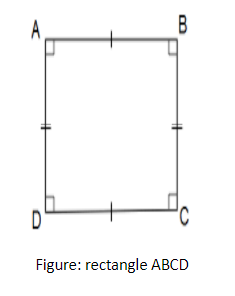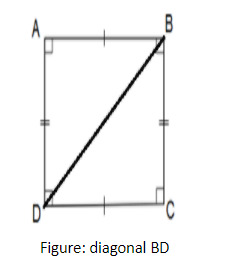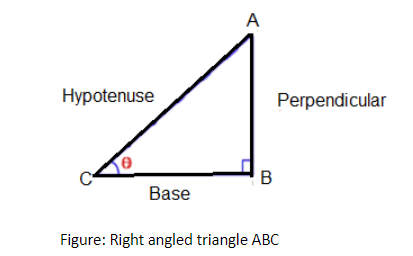The ratio of two unequal sides of a rectangle is 1:2. If the perimeter is 24 cm then the length of the diagonal is:A) $\dfrac{2}{{\sqrt 5 }}cm$B) $\dfrac{4}{{\sqrt 5 }}cm$C) $4\sqrt 5 cm$D) $2\sqrt 5 cm$Verified
147.3k+ views
Hint: Use perimeter to find the length and breadth of the given rectangle. With the length and breadth of the rectangle, diagonal can be easily found by using Pythagoras theorem on the triangle of sides consisting of length, breadth, and diagonal.
We know that the opposite sides of the rectangle are equal. The angle between two adjacent sides is ${90^ \circ }$.
The angle between two adjacent sides of a rectangle is $90^\circ$.
Remember that: Pythagoras theorem can be applied to every right-angled triangle.
Pythagoras theorem: square of the hypotenuse is equal to the sum of the square of base and square of perpendicular.
In a right-angled triangle, base and perpendicular are at the angle of $90^\circ$ to each other and hypotenuse is the longest side.
The comparison of two quantities in terms of ‘how many times’ is known as a ratio.
For example: There are 24 girls and 16 boys in a class. Ratio of the numbers of girls to the numbers of boys $= \dfrac{{24}}{{16}} = \dfrac{3}{2}= 3:2$
Therefore, we can get equivalent ratios by multiplying or dividing the numerator and denominator by the same number.
A ratio can be written as a fraction, thus the ratio $3:17$ can be written as $\dfrac{3}{{17}}$.

Complete step by step solution:
Step 1: Draw the rectangle ABCDProperties of rectangle:
LENGTH: Side $AB$ = side $CD$
BREADTH: Side $BC$ = side $AD$
Side $AB \ne$side $BC$
All the interior angles of the rectangle ABCD $= {90^ \circ }$
Step 2: Given that
Perimeter of rectangle $ABCD = 24cm$
Ratio of two unequal sides of rectangle $ABCD = 1:2$
$\Rightarrow \dfrac{{{\text{length}}}}{{{\text{breadth}}}} = \dfrac{1}{2}$
Step 3: multiply numerator and denominator by $x$
$\Rightarrow \dfrac{{{\text{length}}}}{{{\text{breadth}}}} = \dfrac{x}{{2x}}$
$\Rightarrow \dfrac{{{\text{AB}}}}{{{\text{BC}}}} = \dfrac{x}{{2x}}$ (from step 1)
$\Rightarrow {\text{AB}} = x{\text{ cm}} \\ {\text{ BC}} = 2x{\text{ }}cm \\$
Step 4: find length and breadth of rectangle using parameter of rectangle
Given Parameter of rectangle = 24 cm
$2 \times \left( {{\text{length}} + {\text{breadth}}} \right) = 24$
$\Rightarrow 2 \times \left( {{\text{AB}} + {\text{BC}}} \right) = 24$
On substituting the corresponding values,
$\Rightarrow 2 \times \left( {x + 2x} \right) = 24$
On simplifying expressions,
$\Rightarrow {\text{ }}2\left( {3x} \right) = 24$
$\Rightarrow {\text{ }}6x = 24$
On further simplification, we get
$\Rightarrow {\text{ }}x = \dfrac{{24}}{6}$
$\Rightarrow x = 4cm$
Hence, length $AB = x = 4 cm$
And breadth $BC = 2x = 2(4) = 8 cm$
Step 5: Calculate diagonal BD using Pythagoras theorem
Join the Vertex BD, i.e. diagonal of rectangle ABCD.We know, $\angle BCD = {90^ \circ }$,
$\vartriangle BCD$ is a right angled triangle, right angle at vertex C.
Apply Pythagoras theorem on $\vartriangle BCD$.
i.e. Pythagoras theorem: square of the hypotenuse is equal to the sum of the square of base and square of perpendicular.$\mathop {{\text{Hypotenuse}}}\nolimits^{\text{2}} {\text{ = }}\mathop {{\text{ Base}}}\nolimits^{{\text{2 }}} {\text{ + }}\mathop {{\text{ Perpendicular}}}\nolimits^{\text{2}}$
$\mathop {{\text{BD}}}\nolimits^2 = \mathop {{\text{BC}}}\nolimits^2 + \mathop {{\text{CD}}}\nolimits^2$
On substituting the corresponding values,
$\mathop {{\text{BD}}}\nolimits^2 = \mathop 8\nolimits^2 + \mathop 4\nolimits^2$ ( $\because$AB = CD = 4 cm)
$\mathop { \Rightarrow {\text{BD}}}\nolimits^2 = 64 + 16$
On simplification of the above values,
$\Rightarrow \mathop {{\text{BD}}}\nolimits^2 = 80$
$\Rightarrow {\text{BD = }}\sqrt {80}$
On further simplification, we get
$\Rightarrow {\text{BD = 4}}\sqrt 5$

$\therefore$ The length of the diagonal of a given rectangle is $4\sqrt 5 cm$. The correct option is (C).

Note:
Area of rectangle = length $\times$ breadth sq. units
The diagonal of the rectangle bisects its area.
Students may get confused in labeling hypotenuse, perpendicular, and base sides of the right-angled triangle. Remember that the hypotenuse is the longest side in the right-angled triangle. It is the side opposite to the right angle (or ${90^ \circ }$)
Pythagoras theorem only applies to the right-angled triangle, not to every triangle.